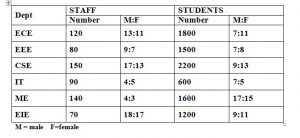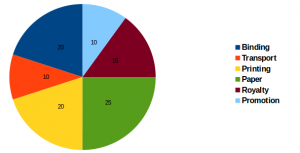## Data Interpretation For SBI PO : Set – 44

D.1-5) Study the following information to answer the given questions1) What is the ratio of total number of staff in ECE,EEE to the total no of students in CSE and IT ?

a) 14:1

b) 1:14

c) 1:12

d) 1:17

e) None of these

b)

Staff = 200

Students = 2800

200: 2800 = 2:28 = 1:14

2) The total no of male staff in IT and EIE is what % of the total number of staff in these 2 dept ?

a) 44.5%

b) 45.7%

c) 47.5%

d) 45.00%

e) None of these

c)

IT = 90 => 4:5 => 40(male)

EIE = 70 => 18:17 => 36(male)

40+36 = 76

Total = 90+70 = 160

76*100/160 = 47.5%

3) Total no of female students in CSE is what % more than the male students in the same Dept ?

a) 44.45%

b) 40.76%

c) 40.75%

d) 40.66%

e) None of these

a)

2200 => 9:13 => 900:1300

400*100/900 = 44.45%

4) Find the number of female students in ME dept ?

a) 850

b) 650

c) 150

d) 750

e) None of these

d)

1600 => 17:15 = 850 : 750(Female)

5) Find the average of total no of staff in all the dept ?

a) 103.8

b) 108.3

c) 108.2

d) 108

e) None of these

b)

(120+80+150+90+140+70)/6 = 650/6 = 108.3

D.6-10) Study the following information to answer the given questions

The values are given in (Percentage)6) If 500 copies are published and the transportation cost on the amount to Rs.2,500 then what should be the selling price of the book so that the publisher can earn a profit of 25%

a) Rs.62.5

b) Rs.65.2

c) Rs.62.2

d) Rs.65.5

e) None of these

a)

10% = 2500

125% = 125*2500/10 = 31250

Sp of 1 book = 31250/500 =62.5

7) If for an edition of the book, the cost of the paper is Rs.6250, then find the promotion cost for this edition ?

a)Rs.2300

b) Rs.4300

c) Rs.2500

d) Rs.2200

e) None of these

c)

25% = 6250

10% = 10*6250/25 = 2500

8) Find the degree corresponding to the Royalty

a) 45degree

b) 23degree

c) 54degree

d) 45degree

e) None of these

C)

R = 15%

Angle = (360*15)/100 = 54degree

9) Which two expenditure together have central angle of144degree

a) Binding And Promotion

b) Transport And Binding

c) Paper And Royalty

d) Paper And Transport

e) None Of These

C)

(x*360)/100 = 144

X = 144*100/360 = 40% = 25%(paper) + 15%(Royalty)

10) Find the total cost to publish 350 book if binding cost is Rs.165

a) 3,89,000

b) 3,98,500

c) 3,58,000

d) 3,40,500

e) 2,88,750